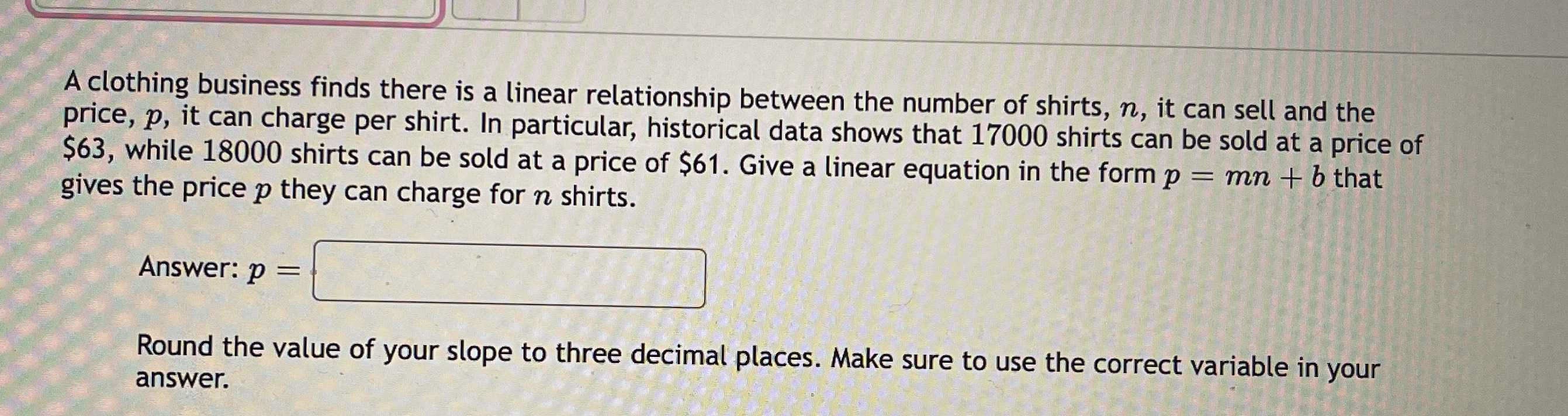### ¿Todavía tienes preguntas de matemáticas?

Pregunte a nuestros tutores expertos
Algebra
PreguntaA clothing business finds there is a linear relationship between the number of shirts, $$n$$ , it can sell and the price, $$p$$ , it can charge per shirt. In particular, historical data shows that $$17000$$ shirts can be sold at a price of $$\ 63$$ , while $$18000$$ shirts can be sold at a price of $$\ 61$$ . Give a linear equation in the form $$p = m n + b$$ that gives the price $$p$$ they can charge for $$n$$ shirts.

Answer: $$p = ?$$

Round the value of your slope to three decimal places. Make sure to use the correct variable in your answer.

$$- 500n+ 48500$$